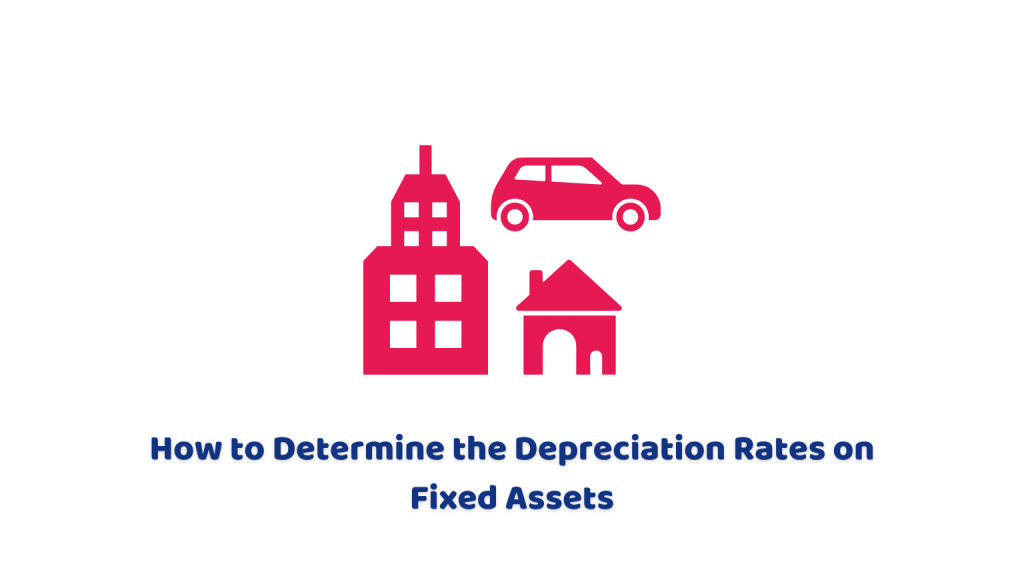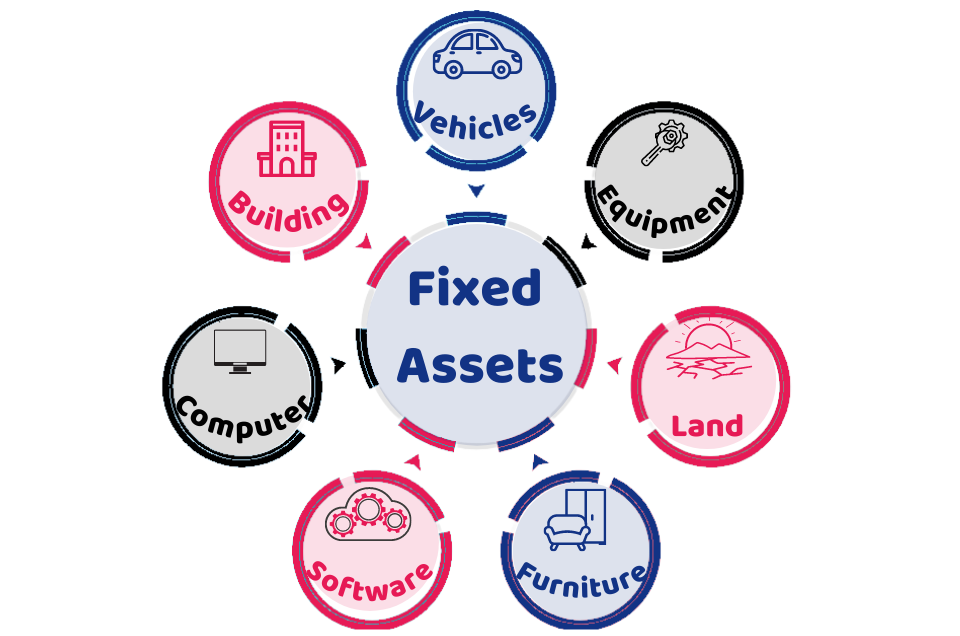# How to Determine the Depreciation Rates on Fixed Assets

Calculating the depreciation of fixed assets has paramount importance to business owners. You can calculate the depreciation of assets in different ways and it varies depending upon your calculation method. This short blog will help you to determine how to calculate the UK depreciation rates on fixed assets of the businesses. Let’s kick off with the basics.

## What is Depreciation?

It is the reduction of the asset’s value due to its usage and wear and tear over a passage of time. In accounting, it is the cost of using an asset over a certain period when it is useful for your business.

## What are Fixed Assets?

Fixed assets are assets having a lifespan of more than one year and used for operating the business. For example, a laptop will be a fixed asset for a freelancer who needs it for running his business.Typically, the fixed asset includes:

• Building
• Computer
• Software
• Furniture
• Land
• Equipment
• Vehicles

## How to Calculate Depreciation?

There are two methods to work out depreciation in the UK: the straight-line method and the reducing balance method. To calculate depreciation, you first need to know the depreciation rates of fixed assets.

Work out your depreciation with our Cheap accountants in London!

## 1. Calculate the UK Depreciation Rates

The depreciation rate is a percentage rate at which the value of the asset decreases in a certain period. Besides, it can also be called the percentage of the long-term investment on an asset that is considered a tax-deductible expense across the useful life of the asset. This rate varies from one asset to another.

### Depreciation Rate Formula

You can calculate the rate of depreciation by this formula:

Depreciation rate per year: useful life an asset in a single year.

Annual Depreciation Value = (Asset’s Cost – Salvage value of Asset)/ Annual Depreciation Rate

Here:

• The cost of an asset refers to the basic value of an asset when someone bought it. It also includes tax and shipping charges, etc.
• The useful life of an asset refers to the lifespan of an asset where it is productive for the business to generate profit. If an asset exceeds its productive life, it will be useless. Like the useful life of a computer is of 5 years and vehicles up to 8 years.
• Savage Value is the scrape value where an asset is no longer useful for a company.

Depreciation rate can be determined based on your asset type and its lifespan. Remember, the depreciation rates of the assets with the residual value will be slightly different.

Importantly, residual value is the value of an asset at the end of its economic life.

However, those assets that increase their value like building land are not depreciated in the Uk accounting.

Here are the typical examples of the depreciation rates of fixed assets:

### The Depreciation rate of Laptop

Suppose, if the lifespan of a Laptop is of 4 years for a freelancer. The rate of depreciation of a laptop will be calculated for 4 years.

### The Depreciation rate of Furniture

If the lifespan of the furniture is of 15 years. You will calculate its depreciation against 15 years.

Still confused! Get in touch with our certified accountants for help.

## 2. Choose a Depreciation Method

There are two methods to work out depreciation:

### Straight Line Method

It is the simplest method to calculate depreciation of the fixed asset by deducting the value of an asset equally each year. Here is the formula:

Depreciation = Cost of Fixed Asset / Useful Life of Fixed Asset

### Reducing Balance Method

If an asset’s value decreases more at the start than at the end, the Reducing Balance Method is used. It is usually implemented on the assets that lose a great value at the start like van etc. Here is the formula:

Depreciation=2×SLDP×BV

Here:

SLDP = Straight-line depreciation percent

BV = Book value at the beginning of the period

• It helps companies to determine the exact market value of the asset
• Depreciation reduces the tax by calculating the decreased value of an asset
• Companies can know their incurred expenses using depreciation

## Quick Wrap Up

To sum up, we can say that depreciation rates on fixed assets vary based on the type of asset and its lifespan as per the income tax department. Using poor methods to calculate depreciation can adversely affect the balance sheet and Profit and loss statement of the company. Therefore, you need to have a firm understanding of these concepts to be on the safe side.

Contact us anytime for any query or help, we’ll get back to you in the shortest time possible!

For a customized package, get an instant quote right away!

Disclaimer: This blog provides general information on Depreciation rates.